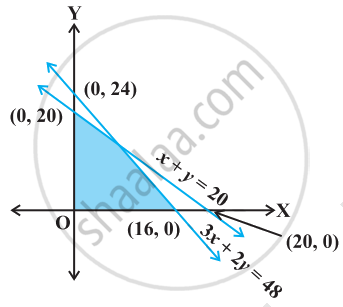# Find the linear inequalities for which the shaded region in the given figure is the solution set. - Mathematics

Sum

Find the linear inequalities for which the shaded region in the given figure is the solution set.#### Solution

Considering 3x + 2y = 48,

The shaded region and the origin both are on the same side of the graph of the line and (0, 0) satisfy the constraint 3x + 2y ≤ 48.

Considering x + y = 20,

The shaded region and the origin both are on the same side of the graph of the line and (0, 0) satisfy the constraint x + y ≤ 20.

We also know that, Shaded region is in the first quadrant i.e. x ≥ 0 and y ≥ 0.

Hence, the linear inequalities are 3x + 2y ≤ 48, x + y ≤ 20, x ≥ 0, y ≥ 0.

Concept: Graphical Solution of Linear Inequalities in Two Variables
Is there an error in this question or solution?

#### APPEARS IN

NCERT Mathematics Exemplar Class 11
Chapter 6 Linear Inequalities
Exercise | Q 14 | Page 108

Share